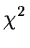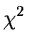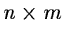Next: Related Table Commands Up: Multivariate Analysis Methods Previous: Discriminant Analysis

Correspondence Analysis

Correspondence Analysis may be described as a PCA in a different metric (themetric replaces the usual Euclidean metric). Mathematically, it differs from PCA also in that points in multidimensional space are considered to have a mass (or weight) associated with them, at their given locations. The percentage inertia explained by axes   takes the place of the percentage variance of PCA, -- and in the former case the values can be so small that such a figure of merit assumes less importance than in the case of PCA. Correspondence Analysis is a technique in which it is a good deal more difficult to interpret results, but it considerably expands the scope of a PCA-type analysis in its ability to handle a wide range of data.

While PCA is particularly suitable for quantitative data, CA is recommendable for the following types of input data, which will subsequently be looked at more closely: frequencies, contingency tables, probabilities, categorical data, and mixed qualitative/categorical data.

In the case of frequencies (i.e. the ijth table entry indicates the frequency of occurrence of attribute j for object i) the row and column profiles'' are of interest. That is to say, the relative magnitudes are of importance. Use of a weighted Euclidean distance, termed thedistance, gives a zero distance for example to the   following 5-coordinate vectors which have identical profiles   of values: (2,7,0,3,1) and (8,28,0,12,4). Probability type values can be constructed here by dividing each value in the vectors by the sum of the respective vector values.

A particular type of frequency of occurrence data is the contingency table, -- a table crossing (usually, two) sets of characteristics of the population under study. As an example, ancontingency table might give frequencies of the existence of n different metals in stars of m different ages. CA allows the study of the two sets of variables which constitute the rows and columns of the contingency table. In its usual variant, PCA would privilege either the rows or the columns by standardizing: if, however, we are dealing with a contingency table, both rows and columns are equally interesting. The standardizing'' inherent in CA (a consequence of thedistance) treats rows and columns in an identical manner. One byproduct is that the row and column projections in the new space may both be plotted on the same output graphic presentations (-- the lack of an analogous direct relationship between row projections and column projections in PCA precludes doing this in the latter technique).

Categorical data may be coded by the scoring'' of 1 (presence) or 0 (absence) for each of the possible categories. Such coding leads to complete disjunctive coding. CA of an array of such complete disjunctive data is referred to as Multiple Correspondence Analysis (MCA) (and in fact such a coding of categorical data is, in fact, closely related to contingency table type data).

Dealing with a complex astronomical catalogue may well give rise in practice to a mixture of quantitative (real valued) and qualitative data. One possibility for the analysis of such data is to discretize'' the quantitative values, and treat them thereafter as categorical. In this way a set of variables -- many more than the initially given set of variables -- which is homogenous, is analysed.Next: Related Table Commands Up: Multivariate Analysis Methods Previous: Discriminant Analysis
Petra Nass
1999-06-15